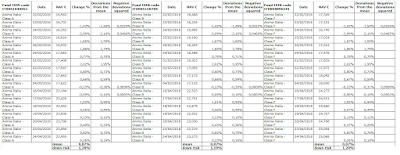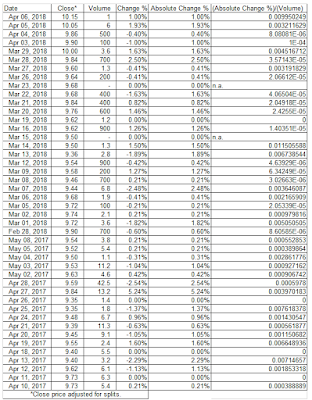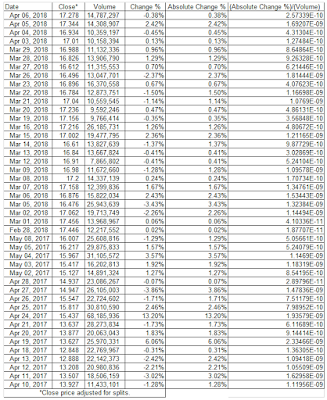## Sunday, April 29, 2018

### The passive management.

The passive management is a style of investing associated with mutual and exchange-traded funds (ETF) where an investor aims to mirror a market index.
We can build the passive strategy through the following steps :
1. Choice of a panel of funds ;
2. Ranking of the funds ;
3. Choice of the funds in the panel with the best ranking.
The panel is chosen in accordance with particular requirements (filter by class, macrocategory, assets, country, risk, currencies and so on ; it depends on the investor's preferences : see the following link ANIMA sgr products).

For example, the aim of the analysis is to rank the funds of the system "Anima Italia" ; the fund ISIN codes are respectively : IT0001040051, IT0005158784, IT0004896541.
The asset allocation is composed by equities, largely ; the currency is EUR ; the equity country is Italy, substantially : for further information, please consult the portfolio breakdown and the fund profile

The site provides a rating and a benchmark for each fund ; however, we can build our benchmark and our rating. For the benchmark, we choose the FTSE-mib index because it can be a good comparative parameter, given the structure of the funds. For the ranking, we use the classic portfolio performance indicators. In this way, we import the NAVs on a excel sheet and then we calculate the daily returns.
The time frame is from February 22, 2016 to date. I converted the daily returns into annual returns, for greater significance.Historical data (hidden cells for space requirements) : data source ANIMA sgr.

The same for the FTSE-mib index, aka benchmark.Historical data : data source Investing.com.

In the following chart, we can see the performance of each fund compared to the benchmark performance.

Now, we can calculate the performance indicators :

• The Sharp's Measure : the ratio uses standard deviation to measure a fund's risk-adjusted returns ; it quantifies a fund's return in excess of our proxy for a risk-free investment. It is equals to :
(R - Rf) / Std Dev
R = average return of the fund ; Std Dev = standard deviation of the fund
Rf = risk-free rate (I assume the average return of the BTP 10Y ITA)

• The Treynor's Measure : the ratio is equal to the previous one ; however, the risk is adjusted for the beta. The index is equal to :
(R - Rf) / Beta
Beta = beta of the fund

• The Jensen's Alpha : the index is a risk-adjusted measure that compares the average return of a fund to the estimated return of the Capital Asset Pricing Model (CAPM). The formula is equal to :
R - [Rf  +  Beta*(Rm - Rf)]
Rm = average return of the benchmark (or market index)

• The M Squared Measure : it is a risk-adjusted measure ; it explains the surplus return of the fund compared to the risk-free investment, considering that the variability of the fund is equal to the variability of the benchmark. The formula is :
(Sharp's Measure)*(Std Devm) + Rf
Std Devm = standard deviation of the benchmark (or market index)

• The T Squared Measure : the structure is the same compared to the previous one ; the difference is the risk, systematic risk or beta ; substantially, it calcualtes the surplus return compared to the risk-free rate, under the assumption that the systematic risk of the fund is equal to the systematic risk of the market. The formula is :
[(1 / Beta)*(R - Rf) - (Rm - Rf)]

• The Sortino Index : rather than considering premiums regarding the risk-free asset, the index explains the surplus return with a minimum accettable return ; then, about the risk, it considers a minimum accettable risk, aka down side risk (the variability not appreciated by the investor ; we calculate a semi-standard deviation, only the negative deviations from the mean). The ratio is equal to :
(R - Minimum Return) / Down Side Risk
For semplicity, we consider the minimum return equal to the risk-free return

Finally, the higher the ratios, the better fund past performance (we must note that the future performance is not linked to the past performance ; however, it is a good beginning).
In this way, we can calculate the ratios and rank the three funds (see the following table).

The ranking is :

1) Fund ISIN code IT0004896541 (the best) ;
2) Fund ISIN code IT0001040051 ;
3) Fund ISIN code IT0005158784 (the worst).

## Monday, April 9, 2018

### The "magnitude" of the liquidity.

In the building of a trading strategy, the liquidity is a crucial variable.
Secondly, the liquidity can be both a positive and a negative factor.
Of course, we have two market scenarios :
1. An illiquid market ;
2. A liquid market.
In the first one, from the negative point of view, the price of a stock hardly represents the intrinsic value : in other words, the stock price will reflect poorly the underlying drivers of the fundamental analysis. The same is also for the technical analysis. The stock price doesn't correctly respond to the graphic signals. This bias can last for a long time and sometimes the gap cannot be solved.
In this way, the market context is artificial.
From the other point of view, we can use that fault to our advantage. That means that it needs to follow the next steps of the market maker. If on one hand, the market maker (aka MM) easily moves the price for lack of liquidity, on the other, we must not suffer this but we must make ourselves part of it.

In the second scenario, there is not the abovementioned bias because the market price responds quickly to the underlying factors (technical or fundamental). However, we cannot use to our advantage the previous fault. The market context is normal.

Finally, the first scenario is difficult to manage than the second one because the illiquid market is difficult to interpret. Neverherless, if we are be able to follow the MM's moves, this will lead to higher profits for the reason that the stock price is much more influential to price changes in the face of low traded volume, due to the market structure.

After this introduction, it is useful to indentify the two scenarios (1. and 2.) and above all the magnitude of the price influenceability.

The following methodology shows that. The steps are :

1. Building the daily returns (%) for a time period (in general, one year is appropriate) ;
2. Building the daily trading volumes ;
3. Pondering the first one with the second one ; in other words, we consider the ratios (the daily returns are as absolute returns ; we are interested in the amplifying effect and we are not interested in the direction of the effect). The formula is :

[Absolute Price Change %] / [Trading Volume]

The Absolute Price Change % is equal to ABS(Price Change %)

Finally, we calculate an average value of the previous ratio (=AVERAGE[Absolute Price Change %] / [Trading Volume]). Then, we multiply the average ratio for different and hypothetical volume levels. In this way, we can get an idea of the influence of volume on stock price (-> we get the hypotethical price changes % for given volumes).

Of course, this represents the market structure and it should not be considered as a correct value a priori. There are many variables that can change the survey data and the past trend is not like the future trend : the trading book and the market environment are some factors.

In the following chart, we have an example of illiquid market. The stock is COVER 50 S.p.A., a classic example of illiquid stock. The daily volumes are low and we can notice that it needs just a small volume to get a considerable price change (for a trading volume of 1K we have a price change % of 3.48%).Daily Returns, from April 10, 2017 to April 6, 2018, hidden cells for space requirements, data source : Yahoo Finance

We can also analyse the abovementioned effect through a regression line.

In the following charts we have an example of liquid market, the stock is UNICREDIT S.p.A.
The daily volumes are huge and in this way it needs a huge volume to get a substantial price change (indeed, for a trading volume of 1K we have a price change % of 7.85189E-07).
For obvious reasons, the slope of the regression line is lower than the previous one.Daily Returns, from April 10, 2017 to April 6, 2018, hidden cells for space requirements, data source : Yahoo Finance

## Sunday, April 1, 2018

### Estimate of the cost of debt.

The calculation of the firm's cost of debt is an important factor. Then, it has a direct application in various areas ; in particular, it is useful :
• to build a DCF model, in order to value a company (it is a variable to estimate the WACC) ;
• to adjust the market multiples (higher the cost of debt, lower the market multiple and viceversa) ;
• to understand the risk of a business and the debt sustenaibility (in other words, the cost of debt is the proxy of the financial structure) ;
• to value the risk of a stock, indirectly.
And on that note, we can analyze the main calculation methods.

I. The cost of debt : the accounting method.

The accounting method is based on balance sheet data. It is easy to estimate but it is also quite prone to errors. It means that if on one hand, the data are readly available, on the other the result is purely static because it doens't consider the perspective scenarios. Indeed, it is advisable to integrate the method with a perspective business plan, focused on the financial structure of the target firm.

According to this method, the cost of debt is equal to the financial expenses divided by the financial debt. There are two options : the first one considers the gross debt minus the cash and cash equivalents (it means that the debt can be paid by the cash ; this hypothesis is not always true) ; the second one considers the gross debt and the interest expenses (this hypothesis is more prudential).

Here we have an example ; the company is Amplifon S.p.A. We can notice the two options. The accounting data are the fiscal years 2017 and 2016.
For further info, please consult the following links :

As shown in the table, the cost of debt is equal to 7,41% if we consider the net debt and it is equal to 4,63% if we consider the gross debt. An important note : the interest expenses are divided by the average debt with the aim to make comparable the two quantities, the item of the income statement ("flow quantity") and the item of the balance sheet ("stock quantity").

Another option of the accounting method is the following : we can also consider an average figure of the cost of debt on several years (3-5 years). In the same way, we can build a perspective table with the estimated financial debt and interest expenses (the next 3-5 years at the place of the past ones).

II. The cost of debt : the relation between the interest coverage ratio and ratings (the fundamental analysis drivers).

We can link the interest coverage ratio (= EBIT/net financial expenses) to the ratings of a sample.
For example, the professor A. Damodaran (see the link,--> Ratings, Spreads and Interest Coverage Ratios, http://pages.stern.nyu.edu/~adamodar/) has built a sample with the rated companies in United States. There are two tables : for large, for smaller and riskier companies (the proxy of the size is the market cap). With the current exchange rate and with the current m. cap, Amplifon belongs to the second group (https://www.investing.com/currencies/eur-usd).Data source : Damodaran Online

The cost of debt is equal to :

Risk-free rate (Rf) + Default Spread (DS)

For Amplifon the DS is equal to 0.90% (the EBIT interest coverage ratio is 7.76 and the market cap is about \$4 billion). For the Risk-free rate (Rf), we can use the annual return of the 10-year T-bond (see the link http://www.stern.nyu.edu/~adamodar/pc/datasets/histretSP.xls ; data source Damodaran Online). For the year 2017, it is equal to 2.80%. In this way, the cost of debt for Amplifon is :

2.80% + 0.90% = 3.70%

As I said, the sample consists of US companies. For a more appropriate sample, of course, it is advisable to use European companies and rate of returns of government bonds, similarly.

Finally, we must remember that the spread that we add to a base rate can be determined by other fundamental ratios (the interest coverage ratio is just one of the drivers). In this way, the discussed methodology is a part of the framework based on the fundamental analysis.

III. The cost of debt : the listed bonds.

The last method is among the most reliable and the easiest to apply methods. If we have a firm with listed bonds, the cost of debt is equal to the rate of return of the mentioned bonds (-->yield to maturity, YTM). Alternatively, we can take as a benchmark a panel of similar listed bonds.

For Amplifon, we can consider the bond listed on the Luxembourg Stock Exchange (LuxSE).
See the following link : https://www.bourse.lu/security/XS0953207759/200201